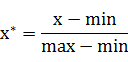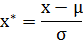# min-max标准化（Min-Max Normalization）min-max标准化python代码如下：

import numpy as np

arr = np.asarray([0, 10, 50, 80, 100])
for x in arr:
x = float(x - np.min(arr))/(np.max(arr)- np.min(arr))
print x

# output
# 0.0
# 0.1
# 0.5
# 0.8
# 1.0


• 1、对于方差非常小的属性可以增强其稳定性；
• 2、维持稀疏矩阵中为0的条目。

from sklearn import preprocessing

import numpy as np

X = np.array([[ 1., -1.,  2.],

[ 2.,  0.,  0.],

[ 0.,  1., -1.]])

min_max_scaler = preprocessing.MinMaxScaler()

X_minMax = min_max_scaler.fit_transform(X)  

array([[ 0.5       ,  0.        ,  1.        ],
[ 1.        ,  0.5       ,  0.33333333],
[ 0.        ,  1.        ,  0.        ]])


X_std=(X-X.min(axis=0))/(X.max(axis=0)-X.min(axis=0))
X_minmax=X_std/(X.max(axis=0)-X.min(axis=0))+X.min(axis=0))

​​​

# Z-score标准化方法import numpy as np

arr = np.asarray([0, 10, 50, 80, 100])
for x in arr:
x = float(x - arr.mean())/arr.std()
print x

# output
# -1.24101045599
# -0.982466610991
# 0.0517087689995
# 0.827340303992
# 1.34442799399


参考来源：点击打开链接

10-191万+
07-21948410-0413万+
10-111621
12-192万+
03-28719
04-156913
03-144964
08-088989
03-096861